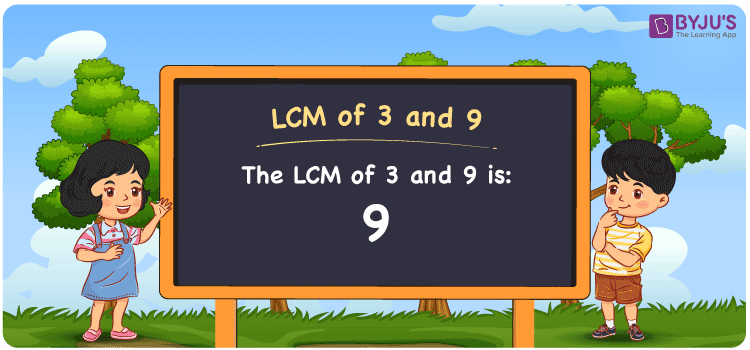Checkout JEE MAINS 2022 Question Paper Analysis : Checkout JEE MAINS 2022 Question Paper Analysis :

# LCM of 3 and 9

LCM of 3 and 9 is 9. The number evenly divisible by the given numbers provides the LCM. From the multiples which are common, the least common multiple of 3 and 9 can be found. The multiples of 3 are (3, 6, 9, 12, 15, 18, 21, ….) and the multiples of 9 are (9, 18, 27, 36, 45, 54, ….) respectively. The methods used to calculate the LCM are prime factorisation, division and by listing the multiples.

## What is LCM of 3 and 9?

The answer to this question is 9. The LCM of 3 and 9 using various methods is shown in this article for your reference. The LCM of two non-zero integers, 3 and 9, is the smallest positive integer 9 which is divisible by both 3 and 9 with no remainder.## How to Find LCM of 3 and 9?

LCM of 3 and 9 can be found using three methods:

• Prime Factorisation
• Division method
• Listing the multiples

### LCM of 3 and 9 Using Prime Factorisation Method

The prime factorisation of 3 and 9, respectively, is given by:

3 = 3¹

9 = 3 x 3 = 3²

LCM (3, 9) = 9

### LCM of 3 and 9 Using Division Method

We’ll divide the numbers (3, 9) by their prime factors to get the LCM of 3 and 9 using the division method (preferably common). The LCM of 3 and 9 is calculated by multiplying these divisors.

 3 3 9 3 1 3 1 1

No further division can be done.

Hence, LCM (3, 9) = 9

### LCM of 3 and 9 Using Listing the Multiples

To calculate the LCM of 3 and 9 by listing out the common multiples, list the multiples as shown below

 Multiples of 3 Multiples of 9 3 9 6 18 9 27 12 36 15 45

LCM (3, 9) = 9

## Video Lesson on Applications of LCM## LCM of 3 and 9 Solved Examples

The LCM and GCD of two numbers are 9 and 3 respectively. If one number is 9, what is the other number?

Solution:

Consider the number as z

We know that

GCD x LCM = 9 x z

z = (GCD x LCM)/9

Substituting the values

z = (9 x 3)/9

z = 3

Hence, the other number is 3.

## Frequently Asked Questions on LCM of 3 and 9

### If the LCM of 3 and 9is 9, what is its GCF?

We know that

LCM x GCF = 3 x 9

As LCM (3, 9) is 9

9 x GCF = 27

GCF = 27/9 = 3

### Find the LCM of 3 and 9.

The LCM of 3 and 9 is 9. To find the LCM, we first should understand the multiples of 3 and 9

Multiples of 3 = 3, 6, 9, 12, 15, 18, ……

Multiples of 9 = 9, 18, 27, 36, 45, 54, ….

The smallest multiple exactly divisible by 3 and 9 is 9.

### Show the relation between GCF and LCM of 3 and 9.

The relation between GCF and LCM of 3 and 9 is

LCM x GCF = 3 x 9

LCM x GCF = 27